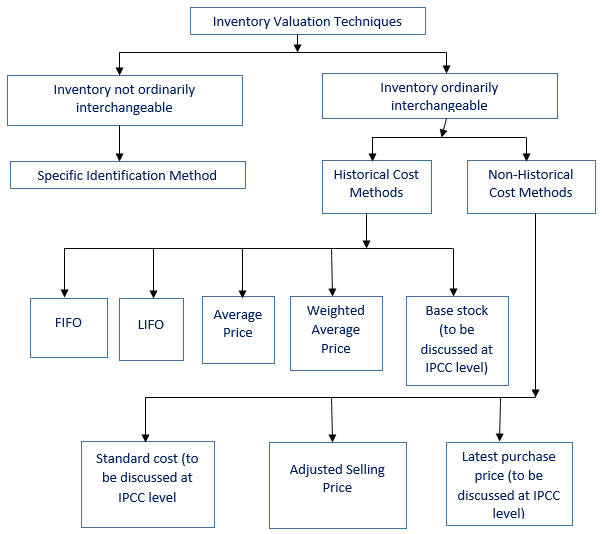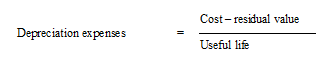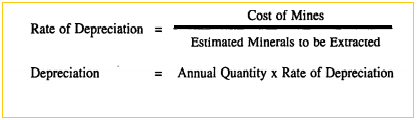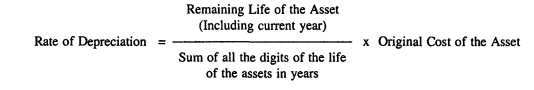Home » Subject » Management » Notes » Inventory valuation and depreciation

# Inventory Valuation

Inventory is current assets and it comprises of major part of financial statement in business and manufacturing concerns (Rajasekaran V., 2011). An inventory valuation is important process which assists company to provide a monetary value for items that comprise their inventory. Inventory includes the products available for resale to customers. Inventories are usually the largest existing asset of a business, and proper measurement of them is required to assure precise financial statements. If inventory is not correctly measured, expenses and revenues cannot be correctly matched and a company could make poor business decisions. The inventory valuation consists of all of the costs to get the inventory items in place and ready for sale. The inventory valuation eliminates the costs of business and management. Inventory valuation is critical to income measurement and inventory management is crucial to financial management. In management accounting, theorists described Inventories as assets that held for sale in the ordinary course of business, in the process of production or manufacture and in the form of materials and supplies to be consumed in such process of such production or manufacture. Therefore inventories refer to finished goods inventory, Work in process inventory, Raw materials, stores and supplies. The closing stock an accounting period will become the opening stock of next period therefore valuation of inventories has more significance. But inventory valuation is not accurate because of policies of management of firms and accounting principles adopted by accountants (Rajasekaran V., 2011).

Significance of inventories: Inventory is major assets for business enterprises. Simple way to judge the liquidity position of enterprise is to compute accounting ratios. Inventory valuation is of great importance in judging of liquidity of concern. Another importance of inventory valuation is that in order to determine true income, proper valuation is necessary (Rajasekaran V., 2011):

Gross profit = sales - cost of sold goods.

Inventory valuation is significant to estimate correct gross profit, for comparison, to determine the correct financial position, for good decision making by management and to estimate next year's purchase (Sripal Jain, 2015).

Valuation of inventories: According to the generally accepted accounting principles, inventories should be measured at cost. Cost refers to cost of acquisition plus cost of conversion.Raw materials, Stores, spare parts, consumables is equal to cost of acquisition that is purchase costs including duties and taxes, freight and other expenses directly related to such purchases. Similarly any discounts, rebates on such purchases should be reduced.

Finished goods in case of manufacturing concern = cost of raw materials plus cost of conversion of raw materials into finished goods. It consists of direct expenses like labour costs as well as indirect manufacturing costs like power. Water, fuel, factory rent, factory insurance etc directly attributable to production / manufacturing. Indirect expenses like salaries, office expenses etc. are not included since they are period costs.

Work in process = WIP is valued at cost as above depending upon the stage of completion of labour and overheads which is determined by the production department.

Inventory Valuation Methods: Even though inventories are to be valued at cost, the cost continuously changes during the year. It may not be always possible or practicable to link the cost of purchase with the closing stock of goods on hand. Inventory valuation methods are used to compute the cost of goods sold and cost of ending inventory. There are numerous inventory valuation at cost methods:

1. First-In, First-Out Method
2. Last-In, First-Out Method
3. Average Cost Method

First-in-First-Out Method (FIFO): This method indicates that items from the inventory are sold in the order in which they are acquired or produced. This indicates that cost of older inventory is charged to cost of goods sold first and the ending inventory consists of those goods which are purchased or produced later. This is the most common method for inventory valuation. This method is nearer to actual physical flow of goods because companies usually sell goods in order in which they are purchased or produced.

Last-in-First-Out Method: It is abbreviated as LIFO. This method of inventory valuation is exactly contrast to first-in-first-out method. In this procedure, it is assumed that newer inventory is sold first and older remains in inventory. When prices of goods increase, cost of goods sold in LIFO method is comparatively higher and ending inventory balance is relatively lower. This is because the cost goods sold mostly consists of newer higher priced goods and ending inventory cost consists of older low priced items.

Average Cost Method: It is called (AVCO). In this method, weighted average cost per unit is calculated for the whole inventory on hand which is used to record cost of goods sold. Weighted average cost per unit is computed as follows:

Weighted Average Cost Per Unit = Total Cost of Goods in Inventory

Total Units in Inventory: The weighted average cost as considered above is multiplied by number of units sold to get cost of goods sold and with number of units in ending inventory to obtain cost of ending inventory.

Figure: Techniques of inventory evaluation ( Source: Sripal Jain, 2015 )It is established that inventory is most significant component of current assets held by manufacturing enterprises. Inventory valuation is needed to determine true income earned by business during a particular period.

## Depreciation

Depreciation is explained as the expensing of the cost of an asset involved in producing revenues all through its useful life. Depreciation in accounting arena denotes to the allocation of the cost of assets to periods in which the assets are used that means depreciation with the matching of revenues to expenses principle. Depreciation expense impacts the values of businesses and entities because the accumulated depreciation revealed for each asset lessen its book value on the balance sheet. Depreciation expense also affects net income. Usually the cost is allocated as depreciation expense among the periods in which the asset is expected to be used. Such expense is documented by businesses for financial reporting and tax purposes.

Generally, depreciation is the diminution in the value of an asset because of usage, passage of time, wear and tear, technological obsolescence, depletion, insufficiency, decay and other numerous factors. It is a fall in the value of an asset. Every fixed asset is liable to loss its value once it is put to use, sooner or later the asset will become useless. Accounting standard 6 describes depreciation as a measure of wearing out, consumption or other losses of value of a depreciable asset arising from use and obsolescence through technology. Pickle explained depreciation as permanent and continuing diminution in quantity, quality or value of an assets (Singhvi, 2006).

As per accounting principles, depreciation is used to explain any method of attributing the past or purchase cost of an asset across its useful life, approximately corresponding to normal wear and tear. It is mainly used when dealing with assets of a short, fixed service life, and which is an example of applying the matching principle as per generally accepted accounting principles. Depreciation is computed on all depreciable possessions which can be classified as those which have a useful life for more than one accounting period but is restricted and are held by an enterprise for use in the production or supply of goods and services. Many examples of depreciable assets can be sited such as machines, plants, furniture, buildings, computers, trucks, vans, equipment. Moreover, depreciation is the allocation of 'depreciable amount' which is the 'historical cost' or other amount substituted for historical cost less estimated salvage value. Depreciation has noteworthy effect to assess and present the monetary position and results of operations of an enterprise. Depreciation is charged in each accounting period by reference to the extent of the depreciable amount. A systematic process for allocating the cost over the periods of its useful life in a logical manner is termed as depreciation accounting.

## Features of Depreciation

There are numerous features of depreciation (Singhvi, 2006):

1. Depreciation reduces the book value of fixed assets but not its market value.
2. Depreciation includes loss of value of assets due to passage of time, usage or obsolescence.
3. Depreciation is a continuing process till the end of the useful life of assets.
4. Depreciation is an expired cost and hence must be deducted before calculating taxable profits.
5. Depreciation is a non-cash expense. It does not involve any cash flow.
6. Depreciation is the process of writing-off the capital expenditure already incurred.
7. It is a measure of loss of value of asset.
8. The phrase depreciation is only used for tangible fixed assets.

Main causes of depreciation: The causes of depreciation are as follows (Singhvi, 2006):

1. Wear and tear: It is an important cause of depreciation in case of tangible fixed assets. It is mainly due to use of assets.
2. Obsolescence: Due to technology up gradation, value of assets decreases. If better machine introduced in market, the old one may be scrapped even through it is capable of producing.
3. Accidents: Accidental loss may be permanent but it may not be continuing and gradual.

## Purpose of Charging Depreciation

The following are the purpose of charging depreciation of fixed assets:

1. To determine in the true profit of the business.
2. To demonstrate the true presentation of financial position.
3. To offer fund for replacement of assets.
4. To demonstrate the assets at its practical value in the balance sheet.

## Factors Affecting the Amount of Depreciation

Numerous factors must be taken into account when charging the amount of depreciation:

1. The original cost of the asset.
2. The useful life of the asset.
3. Estimated scrap or residual value of the asset at the end of its life.
4. Selecting an appropriate method of depreciation.

## Method of Calculating Depreciation

Various methods are adopted for measuring allocation of depreciation cost:

1. Straight Line Method: This method is also known as Constant Charge Method in which depreciation is charged for every year and it will be the constant amount throughout the life of the asset. Accordingly depreciation is calculated by deducting the scrap value from the original cost of an asset and the balance is divided by the number of years estimated as the life of the asset. The depreciation charge is calculated as (Kolitz, 2009):The major advantage of this procedure is that it is simple and easy to calculate, original cost of asset reduced up to Scrap Value at the end of estimated life and estimated useful life of the asset can be estimated under this method. At the same time, there are some limitations for this method such as it does not consider intensity of use of assets, it disregards any additions or opportunity cost while calculating depreciations, it ignores effective utilization of fixed assets, it becomes difficult to calculate correct depreciation rate and under the assumption of constant charges of maintenance of assets it is impossible to calculate true depreciation.

2. Written Down Value Method: This method is also termed as Fixed Percentage On Declining Base Method or Reducing Instalment Method. In this method, depreciation is charged at fixed rate on the reducing balance, that is, Cost, less depreciation every year. Accordingly the amount of depreciation gradually reduces every year. This method has many benefits such as it is accepted by income tax authorities. Impact of obsolescence will be reduced at minimum level. Fresh calculation is not required when additions are made. Under this method, the depreciation amount is gradually decreasing and it will affect the smoothing out of periodic profit. Disadvantages of this procedure are Residual Value of the asset cannot be correctly estimated, it ignores interest on investment on opportunity cost which will lead to difficulty while determining the rate of depreciation. It is difficult to ascertain the true profit because revenue contribution of the asset are not constant. The original cost of the asset cannot be brought down to zero.
3. Annuity Method: This method is most appropriate for enterprises where capital is invested in the least hold properties. Under this method, while calculating the amount of depreciation, a fixed amount of depreciation is charged for every year of the estimated useful life of the asset in such a way that at a fixed rate of interest is calculated on the same amount had been invested in some other form of capital investment. In other words, depreciation is charged for every year refers to interest losing or reduction in the original cost of the fixed assets. Under the annuity method where the loss of interest is due to the investment made in the form of an asset is considered while calculating the depreciation.
4. Sinking Fund Method: The amount of depreciation is charged with the help of Sinking Fund Table. Under this method, an amount equal to the amount written off as depreciation is invested in outside securities in order to facilitate to replace the asset at the expiry useful life of the asset. It can be said that the amount of depreciation charged is debited to depreciation account and an equal amount is credited to Sinking Fund Account. At the estimated expiry useful life of the asset, the amount of depreciation each year is invested in easily realizable securities which can be readily available for the replacement of the asset.
5. Revaluation or Appraisal Method: This method is particularly made to revalue the assets in the case of livestock, loose tools, and patents. This method is also known as Appraisal Method. The computation of depreciation of these assets is valued at the end of the accounting year by comparing the opening value of the asset of the additional if any; the difference is treated as depreciation.
6. Insurance Policy Method: In this method of calculating depreciation, an asset to be replaced by taking required amount of insurance policy from an Insurance Company. A fixed premium is paid which is equal to the amount of depreciation for every year. At the end of the agreed sum, i.e., on the maturity of the policy, the amount is used for replacing the existing assets.
7. Depletion Method: This Method is most suited for natural resources such as mines, quarries, oil and gas from which certain quantity of the resources can be obtained on the basis of the availability of minerals. The quantity of output exhaust to reach a stage of depletion. The rate of depreciation is established on the basis of the quantity obtained for every year. The formula is as under:8. Sum of the Digits Method: This method of computing depreciation is also termed as SYD Method. It is developed on the basis of Written-Down Value Method. Under this method the amount of depreciation to be charged to the Profit and Loss Account goes on decreasing every year throughout the life of the asset. The formula for calculating the amount of depreciation is as follows:9. Machine Hour Rate Method: This method is parallel to the Depletion Method but instead of taking estimated available quantities in advance, the working life of the machine is estimated in terms of hours. The hourly rate of depreciation is determined by dividing the cost of the machine minus scrap value of the machine by the estimated total number of hours utilized every year.

It can be demonstrated in accounting literature that Depreciation is methodical allocation of the cost of a fixed asset over its useful life. Depreciation is part of the original purchase price of the fixed asset consumed during its period of use by the firm. Since the revenue generated from the use of fixed asset will be decreased each year, depreciation thus should be treated as an expense, i.e. it reduces net profit. It is a way of matching the cost of a fixed asset with the revenue it generates over its useful life. Without depreciation accounting, the whole cost of a fixed asset will be documented in the year of purchase. This will give a deceptive view of the profitability of the entity.